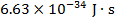# Can a wavelength be smaller than a Planck length?

• B
• sqljunkey
That's right. A particle can move at speeds arbitrarily close to ##c## so there is no cap.But then because an electron can not move at c or we move at c the shortness of the wavelength would be capped at something near c.The electron's speed can be arbitrarily close to ##c## so there is no limit on how short the wavelength can be. As you get closer and closer to speed ##c## it becomes inconvenient to speak of speeds, and so researchers speak about the energy. There is an upper limit on speed, but there is no upper limit on energy.The wavelength of the molecule noted here must be incredibly tinyhttps://f
Plank length? That's about three meters, right?

And there is nothing magic about the Planck length. It's not a quantum of anything or the minimum of anything.

•Ibix
https://en.wikipedia.org/wiki/Planck_length according to this link it is 1.616255(18)×10−35 meters.

and according to the same link, "The Planck length is the scale at which quantum gravitational effects are believed to begin to be apparent, where interactions require a working theory of quantum gravity to be analyzed. The Planck area is the area by which the surface of a spherical black hole increases when the black hole swallows one bit of https://en.wikipedia.org/wiki/Physical_information.[dubiousdiscuss] To measure anything the size of Planck length, the photon momentum needs to be very large due to Heisenberg's uncertainty principle and so much energy in such a small space would create a tiny black hole with the diameter of its event horizon equal to a Planck length."

If I kept increasing the mass of a particle and kept it's speed at .9c would I be able to create wavelengths smaller than the plank length?
Or you could keep its mass the same, don’t worry about its speed, and just increase your speed relative to it. Your speed relative to it can be arbitrarily close to ##c## (you started with ##.9c## here) and then its calculated wavelength will be arbitrarily small.

In any case, the Planck length is just a length, not some physical lower limit on sizes. You may want to take a look at our Insights article on the subject: https://www.physicsforums.com/insights/hand-wavy-discussion-planck-length/

Okay. But then because an electron can not move at c or we move at c the shortness of the wavelength would be capped at something near c. It will take something more massive, such as a proton to reach shorter wavelengths than whatever an electron can ever reach. Right?

But then because an electron can not move at c or we move at c the shortness of the wavelength would be capped at something near c. It will take something more massive, such as a proton to reach shorter wavelengths than whatever an electron can ever reach. Right?
Not right. A particle can move at speeds arbitrarily close to ##c## so there is no cap.

But then because an electron can not move at c or we move at c the shortness of the wavelength would be capped at something near c.
The electron's speed can be arbitrarily close to ##c## so there is no limit on how short the wavelength can be. As you get closer and closer to speed ##c## it becomes inconvenient to speak of speeds, and so researchers speak about the energy. There is an upper limit on speed, but there is no upper limit on energy.

The wavelength of the molecule noted here must be incredibly tiny
https://www.nature.com/articles/ncomms1263 given that de Broglie's equation applieshttps://en.wikipedia.org/wiki/Wave–particle_dualityhttp://www.softschools.com/formulas/physics/de_broglie_wavelength_formula/150/where p is momentum -- mass x velocity
Shorter wavelengths do give better resolution -- but they ALSO impart more energy to the area of the target impacted.
Think of it this way.
You want to explore the shape of a statue by throwing objects of known mass, radius and momentum at it and recording the rebounds. Medicine balls are very heavy and slow with large radii--and give you some data (large mass and radii, small velocity, small p). Basketballs are smaller, less heavy, but can be thrown faster, giving you better data (smaller mass, larger velocity, smaller radii, larger p). Golf balls are smaller and lighter still and can be thrown relatively faster (very small mass and radii but high velocity, so very high p) Bullets are the next step up -- but the bullet doesn't survive the collision to be recorded and the statue doesn't survive being shot at.

That becomes the issue. The more massive and faster the object you throw, the smaller wavelength it has () -- but wavelength and frequency are related inversely (λ = vf) and energy is given by (), so the energy it has increases as the wavelength decreases (infrared < Visible< UV< Gamma< cosmic rays)
Eventually the energy applied destroys the sample.

Now, proton and neutron probes can be useful because their mass limits scattering (think of how a baseball would rebound relatively well from your statue, where ping pong balls would scatter, be overwhelmed by air resistance and generally provide data so noisy as to be useless.)
But they aren't necessarily useful because they have smaller wavelengths.

Now, to get a wavelength less than
Plank Length 1.616255(18)×10−35m
where h = Planck's constant ()
inwould take a p of 0.0244 kg*m/s
The molecule noted in the Nature article weighs 1.14743e-23 kg.
You can do the math on how fast you'd have to whistle even that massive thing to get to 0.0244 kg*m/s
You can also do the math on 0.9c and derive a theoretical rest mass -- but its going to be titanic!